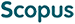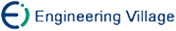张建华, 曾建潮. 基于序贯重点采样粒子滤波和Cholesky分解的分布估计算法[J]. 计算机研究与发展, 2010, 47(11): 1978-1985.
 引用本文: 张建华, 曾建潮. 基于序贯重点采样粒子滤波和Cholesky分解的分布估计算法[J]. 计算机研究与发展, 2010, 47(11): 1978-1985.Zhang Jianhua, Zeng Jianchao. Estimation of Distribution Algorithm Based on Sequential Importance Sampling Particle Filters and Cholesky Decomposition[J]. Journal of Computer Research and Development, 2010, 47(11): 1978-1985.
 Citation: Zhang Jianhua, Zeng Jianchao. Estimation of Distribution Algorithm Based on Sequential Importance Sampling Particle Filters and Cholesky Decomposition[J]. Journal of Computer Research and Development, 2010, 47(11): 1978-1985.## Estimation of Distribution Algorithm Based on Sequential Importance Sampling Particle Filters and Cholesky Decomposition

• 摘要: 连续域分布估计算法一般假设数据服从Gauss分布,而且大多采用了单峰的概率模型,但是对于一些复杂的优化问题,单峰的Gauss分布模型不能有效地描述解在空间的分布.提出一种基于序贯重点采样粒子滤波的分布估计算法,采用带权粒子描述优选集样本服从的概率分布,Cholesky分解法分解收缩的协方差矩阵并利用其产生下一代样本,不需要假设样本服从Gauss分布.算法采用的概率模型是多峰的.变量之间的相关性通过采样时利用群体的协方差矩阵显式地予以考虑,并对协方差矩阵为零矩阵的情况进行了处理.仿真实验结果验证了方法的正确性和有效性.

Abstract: Estimation of distribution algorithm is a new intelligent stochastic optimization algorithm. It is more effectively to solve the non-linear, variable coupling optimization problem. Estimation of distribution algorithm in continuous domains is generally based on the assumptions that variables subject to Gauss distribution and the probability model is single-peaked one, which is not capable of describing the solutions distribution effectively for complex optimization problems. Aiming to overcome such drawbacks, an estimation of distribution algorithm depending upon sequential importance sampling particle filters is presented. In this algorithm, the variables are not required to subject to Gauss distribution. Instead, the distribution of samples is represented by weighted particles through the particle filter iteration on selection set of population. The probability model of this algorithm is multi-peaked and the relations among each dimension are handled using the covariance matrix. In sampling, the covariance matrix is shrunken for each particle and the shrunken covariance matrix is decomposed using Cholesky decomposition, and a method of handling the situation that the covariance matrix is zero matrix is presented. The next generation of population is produced from the decomposition result. Finally, the experimental results of several benchmark functions for complex optimization problems indicate the validity of the algorithm./下载:  全尺寸图片 幻灯片
• 分享
• 用微信扫码二维码

分享至好友和朋友圈